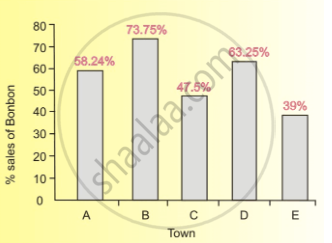# What Was the Total Consumption of Bonbon Bread in Towns a and B During a Particular Week When the Total Consumption of Bread in Town D Was 3600 Loaves? - Mathematics

#### Question

MCQ

Direction:  Answer the question on the basis of the information given below.

The following information is about the consumption of bread in five towns - A, B, C, D and E. Town A consumes a quarter of the total quantity of bread sold in all the five cities. The bread consumption of Town B is one-fourth of the consumption of Town C. The bread consumption of Town D is 12% of the total whereas Town E consumes 23% of the total.

There are only two companies - Bonbon and Modern - that sell bread in these five towns. The bar graph shows the sales of Bon-bon as a percentage of the total sale of bread in each city.What was the total consumption of Bonbon bread in Towns A and B during a particular week when the total consumption of bread in Town D was 3600 loaves?

• 5144

• 6138

• 7605

• 4368

#### Solution

6138

Explanation:

The correct option is (2). According to the information given, The total consumption of bread in Town D was 12% which is equivalent to 3600 loaves. Therefore, the total consumption of bread in Town A can be calculated as:

3600/12 xx 25 = 7500 loaves and the total consumption of bread in Town B can be calculated as :

3600/12 xx 8 = 2400

The consumption of Bonbon bread in Town A

58.24/100 xx 7500 = 4368

and the consumption of Bonbon bread in Town B

73.75/100 xx 2400 = 1770

Hence, total consumption of Bonbon bread in Town A and Town B is (4368 + 1770) = 6138 loaves.

Concept: Graph and Charts (Entrance Exam)
Is there an error in this question or solution?